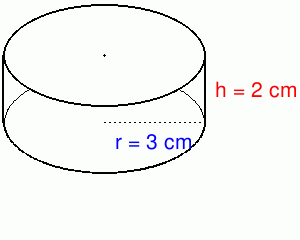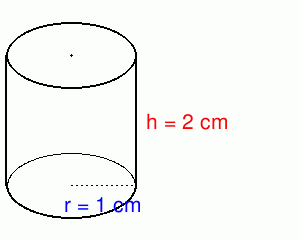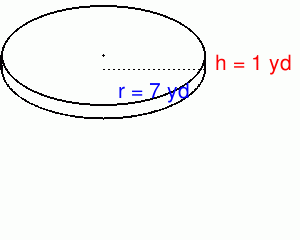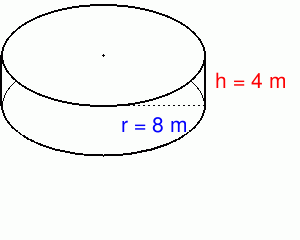MathScore EduFighter is one of the best math games on the Internet today. You can start playing for free!

## Cylinders - Sample Math Practice Problems

The math problems below can be generated by MathScore.com, a math practice program for schools and individual families. References to complexity and mode refer to the overall difficulty of the problems as they appear in the main program. In the main program, all problems are automatically graded and the difficulty adapts dynamically based on performance. Answers to these sample questions appear at the bottom of the page. This page does not grade your responses.

See some of our other supported math practice problems.

### Complexity=1

Find the surface area and volume. Answer with proper units like m2 and m3.
To represent m2, use "sq m", etc. To answer with π, use "pi".
Sample area: 5π m2 becomes "5pi sq m". Sample volume: 5π m3 becomes "5pi cu m".

 1Surface Area = Volume = 2Surface Area = Volume =

### Complexity=2

Find the surface area and volume. Answer with proper units like m2 and m3.
To represent m2, use "sq m", etc. To answer with π, use "pi".
Sample area: 5π m2 becomes "5pi sq m". Sample volume: 5π m3 becomes "5pi cu m".

 1Surface Area = Volume = 2Surface Area = Volume =

### Complexity=1

Find the surface area and volume. Answer with proper units like m2 and m3.
To represent m2, use "sq m", etc. To answer with π, use "pi".
Sample area: 5π m2 becomes "5pi sq m". Sample volume: 5π m3 becomes "5pi cu m".

1Surface Area =
Volume =
Solution
The surface area of a cylinder is the area of the bottom and top circles and the area of the side of the cylinder. This can be expressed as follows:
SA = 2πrh + 2πr2
SA = 2π × (3cm) × (2 cm) + 2π × (3 cm)2
SA = 2π × (6 cm2) + 2π × (9 cm2)
SA = 12π cm2 + 18π cm2
SA = 30π cm2

The volume of a cylinder is the area of the base circle times the height of the cylinder.
V = bh = πr2h
V = π × (3 cm)2 × (2 cm)
V = π × (9 cm2) × (2 cm)
V = π × (18 cm3)
V = 18π cm3
2Surface Area =
Volume =
Solution
The surface area of a cylinder is the area of the bottom and top circles and the area of the side of the cylinder. This can be expressed as follows:
SA = 2πrh + 2πr2
SA = 2π × (1cm) × (2 cm) + 2π × (1 cm)2
SA = 2π × (2 cm2) + 2π × (1 cm2)
SA = 4π cm2 + 2π cm2
SA = 6π cm2

The volume of a cylinder is the area of the base circle times the height of the cylinder.
V = bh = πr2h
V = π × (1 cm)2 × (2 cm)
V = π × (1 cm2) × (2 cm)
V = π × (2 cm3)
V = 2π cm3

### Complexity=2

Find the surface area and volume. Answer with proper units like m2 and m3.
To represent m2, use "sq m", etc. To answer with π, use "pi".
Sample area: 5π m2 becomes "5pi sq m". Sample volume: 5π m3 becomes "5pi cu m".

1Surface Area =
Volume =
Solution
The surface area of a cylinder is the area of the bottom and top circles and the area of the side of the cylinder. This can be expressed as follows:
SA = 2πrh + 2πr2
SA = 2π × (7yd) × (1 yd) + 2π × (7 yd)2
SA = 2π × (7 yd2) + 2π × (49 yd2)
SA = 14π yd2 + 98π yd2
SA = 112π yd2

The volume of a cylinder is the area of the base circle times the height of the cylinder.
V = bh = πr2h
V = π × (7 yd)2 × (1 yd)
V = π × (49 yd2) × (1 yd)
V = π × (49 yd3)
V = 49π yd3
2Surface Area =
Volume =
Solution
The surface area of a cylinder is the area of the bottom and top circles and the area of the side of the cylinder. This can be expressed as follows:
SA = 2πrh + 2πr2
SA = 2π × (8m) × (4 m) + 2π × (8 m)2
SA = 2π × (32 m2) + 2π × (64 m2)
SA = 64π m2 + 128π m2
SA = 192π m2

The volume of a cylinder is the area of the base circle times the height of the cylinder.
V = bh = πr2h
V = π × (8 m)2 × (4 m)
V = π × (64 m2) × (4 m)
V = π × (256 m3)
V = 256π m3